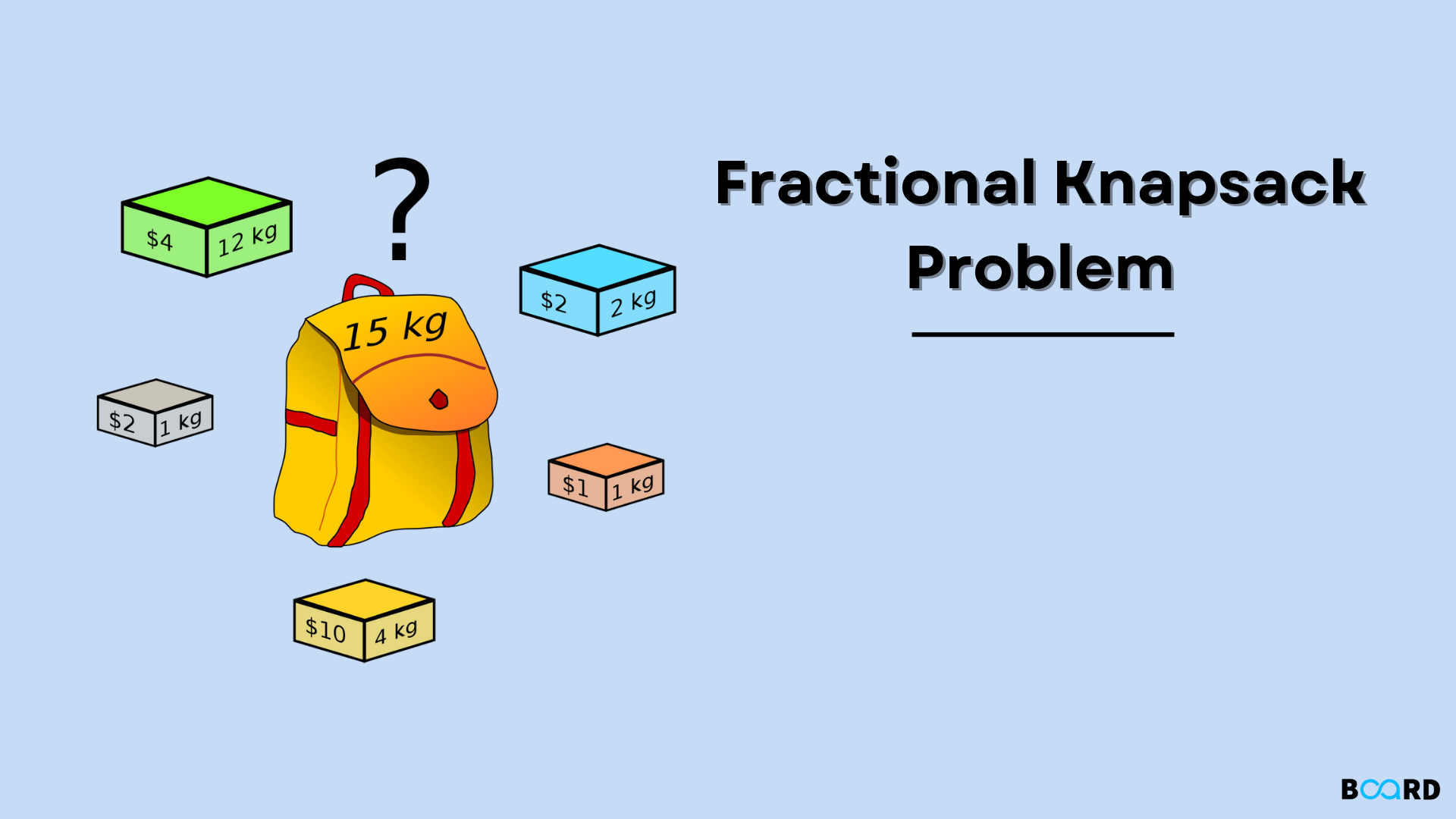# Fractional Knapsack Problem: Greedy Approach## Problem Statement

Given the weights W and values V of N items which are to be inserted in the knapsack which can bear the weight upto K. Insert maximum value in the knapsack with weight not greater than K. Every item can be inserted in fractional manner also( i.e, we can break the iteam to maximise the value in the knapsack).

## Examples

For better understanding of the problem statment, lets look at the below example:

Input: arr={(20,4),(63,7),(40,8),(30,5)} and K= 18

Explanation: Array is of format {(Value,Weight)}. To maximise the value in the bag with weight not more than K and also breaking of items is allowed. So, we can use a greedy approach in this problem. Firstly, find the ratio of Value/Weight  and using this ratio. Arrange the items to maximise the profit. The ratios of the given input in the descending  order is as follows:

 Item 2(W/V) Item 4(W/V) Item 1(W/V) Item 3(W/V) 63/7=9 30/5=6 20/4=5 40/8=5

The knapsack capacity is given as K= 18. So, accordingly we can fit upto:

Item 2 weight +Item 4 weight + Item 1 weight + 1/4(Item 3 weight)= 18

Therefore. The value of the knapsack is 63+30+20+¼(40)= 123

Output: 123

## Greedy Approach

In the above example, we have discussed to obtain the maximum value in the knapsack. We need to find the ratio of Value/Weight  and we need to return the maximum value of the knapsack obtained. Finding all the ratios and arranging them in descending order and adding into the knapsack without exceeding the capacity of the knapsack. This is performed greedily by breaking the iteams whenever needed. This can be clearly explained in the below implemented code:

 # Python Program for fractional knapsack # Class which defined the structure of an item which stores weight and  corresponding value of item from the given inputclass Item:    def __init__(self, value, weight):        # constructor initialisation        self.value = value        self.weight = weight # Greedy function for finding the maximum value in the knapsack.def fractionalKnapsack(K, arr):     # Sorting Item on basis of ratio in descending order    arr.sort(key=lambda x: (x.value/x.weight), reverse=True)       # Final value in knapsack     Value_Knapsack = 0.0     # Looping through all Items such that knapsack doesnt exceed its capacity     for i in arr:         # If adding Item in the knapsack doesnt exceed the capacity add it in the knapsack else return the final value        if i.weight <= K:            K -= i.weight            Value_Knapsack += i.value         # If we can't add current Item completely, add the fractional value until it does not exceed the capacity K        else:            Value_Knapsack += i.value * K / i.weight            break         # Returning final value of the knapsack     return Value_Knapsack # Driver Code# Capacity of the knapsackK = 18# input weight and value array arr = [Item(20, 4), Item(63, 7), Item(40, 8), Item(30,5)] # Greedy funciton is called to obtain the final valueans = fractionalKnapsack(K, arr)print(ans)
write your code here: Coding Playground

## Complexity Analysis

Time Complexity: O( N*LogN), where N is the number of items given.

Finding the ratio and sorting them in the descending order using merge sort, has the overall time complexity of O(N* Log N).

Space Compleity: O(N), where N is the number of items given.

Extra space is needed during the merge sort for arranging the ratios in descending order.

## Conclusion

In the fractional knapsack, to maimise the value in the knapsack by not exceeding the capacity of the knapsack is resolved using a greedy approach of time complexity O(N LogN) and space complexity of O(N).